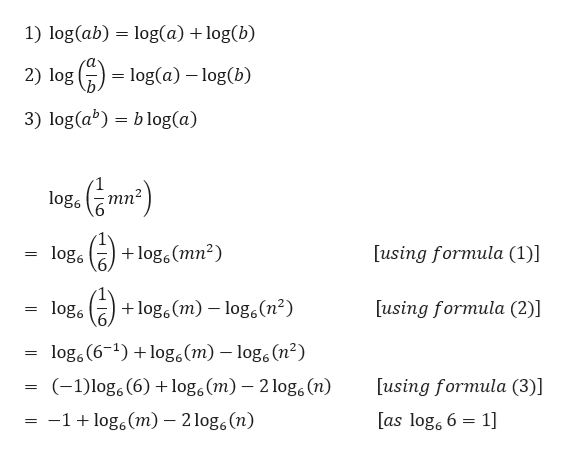# Write the logarithm as a sum or difference of logarithms. Simplify each term as much as possible. Assume that the variables represent positive real numbers.Log6 (1/6 mn2)

Question
34 views

Write the logarithm as a sum or difference of logarithms. Simplify each term as much as possible. Assume that the variables represent positive real numbers.

Log6 (1/6 mn2)

check_circle

Step 1

We need to simplify the given logarithm

Step 2

We know th...help_outlineImage Transcriptionclose1) log(ab) = log(a) + log(b) 2) log (G) = log(a) – log(b) 3) log(a) = b log(a) log. (e mr) log. ) +log.(mn²) [using formula (1)] + log. (m) – log.(n²) [using formula (2)] log. log, (6-1) +log.(m) – log. (n²) (-1)log, (6) +log.(m) – 2 log, (n) [using formula (3)] %3D [as log, 6 = 1] -1+ log.(m) – 2 log,(n) fullscreen

### Want to see the full answer?

See Solution

#### Want to see this answer and more?

Solutions are written by subject experts who are available 24/7. Questions are typically answered within 1 hour.*

See Solution
*Response times may vary by subject and question.
Tagged in
MathAlgebra

### Logarithms